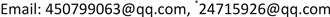﻿ 基于均差估计二变量Meta-分析的未知相等方差模型 The Unknown Equal Variance Model of Bivariate Meta-Analysis Based on the Difference Mean

Statistics and Application
Vol. 08  No. 01 ( 2019 ), Article ID: 28829 , 9 pages
10.12677/SA.2019.81015

The Unknown Equal Variance Model of Bivariate Meta-Analysis Based on the Difference Mean

Pengfei Guo1,2,3, Shuangyin Liu2,3,4, Gang Li5*, Lei Zhang2,3,4, Liang Cao2,3,4, Xiangru Sun6, Longqin Xu2,3,4, Qian Wang1

1College of Computational Science, Zhongkai University of Agriculture and Engineering, Guangzhou Guangdong

2Intelligent Agriculture Engineering Research Center of Guangdong Higher Education Institutes, Zhongkai University of Agriculture and Engineering, Guangzhou Guangdong

3Guangdong Province Key Laboratory of Waterfowl Healthy Breeding, Zhongkai University of Agriculture and Engineering, Guangzhou Guangdong

4College of Information Science and Technology, Zhongkai University of Agriculture and Engineering, Guangzhou Guangdong

5Institute of Reproductive Medicine, Panyu Hexian Memorial Hospital of Guangzhou, Guangzhou Guangdong

6Guangzhou E-Government Center, Guangzhou GuangdongReceived: Jan. 24th, 2019; accepted: Feb. 6th, 2019; published: Feb. 13th, 2019ABSTRACT

This paper introduces the unknown equal variance model of bivariate Meta-analysis based on the difference mean. We obtain the maximum likelihood estimator of the difference mean effect and unknown variances; then we find the combining overall difference mean effect ${D}_{overall}$ by the fixed-effect model of bivariate Meta-analysis based on the difference mean. Moreover, we obtain the corresponding covariance matrix and the $\left(1-\alpha \right)100%$ confidence interval for the overall difference mean effect
${D}_{overall}$ .

Keywords:Meta-Analysis, The Maximum Likelihood Estimate, Fixed-Effect Model1仲恺农业工程学院计算科学学院，广东 广州

2仲恺农业工程学院广东省高校智慧农业工程研究中心，广东 广州

3仲恺农业工程学院广东省水禽健康养殖重点实验室，广东 广州

4仲恺农业工程学院信息科学与技术学院，广东 广州

5广州市电子政务服务中心，广东 广州

6番禺何贤纪念医院生殖医学科，广东 广州Copyright © 2019 by author(s) and Hans Publishers Inc.1. 引言

Meta-分析是针对同一问题的不同研究进行定量合并的综合评价方法。虽然Meta-分析在很多应用领域都有较好的应用，但对Meta-分析本身的统计方法研究相对少了很多。从广义上来讲，Meta-分析是以估计量标准误差的逆为权重的点估计加权平均。固定效应模型Meta-分析依赖于各个研究具有相等效应量，最终的效应量是以估计量方差的逆作为权重得到的加权平均效应量。虽然固定效应模型变量之间不允许有协变量，但是对固定响应模型的处理相对来说是很标准的。

2. 问题描述

${D}_{i}=\left(\begin{array}{c}{D}_{i1}\\ {D}_{i2}\end{array}\right)\sim N\left(\left(\begin{array}{c}{\mu }_{1}\\ {\mu }_{2}\end{array}\right),\left(\begin{array}{cc}{\sigma }^{\text{2}}{w}_{i1}& \text{0}\\ \text{0}& {\tau }^{2}{w}_{i2}\end{array}\right)\right)$

3. 均值效应量的极大似然估计

$L\left(\mu ,{D}_{i},{S}_{i}\right)=\underset{i=1}{\overset{k}{\prod }}\frac{1}{2\text{π}det{\left({S}_{i}\right)}^{\frac{1}{2}}}\mathrm{exp}\left\{-\frac{{\left({D}_{i}-\mu \right)}^{\text{T}}{S}_{i}^{-1}\left({D}_{i}-\mu \right)}{2}\right\}$

$\mathrm{ln}L\left(\mu ,{D}_{i},{S}_{i}\right)=-k\mathrm{ln}\left(\text{2π}\right)-\frac{1}{2}\underset{i=1}{\overset{k}{\sum }}\mathrm{ln}\left[det\left({S}_{i}\right)\right]-\frac{1}{2}\underset{i=1}{\overset{k}{\sum }}{\left({D}_{i}-\mu \right)}^{\text{T}}{S}_{i}^{-1}\left({D}_{i}-\mu \right)$

1). $\mu$ 的极大似然估计量的分量为： ${\stackrel{^}{\mu }}_{1}=\frac{\underset{i=1}{\overset{k}{\sum }}\frac{{D}_{i1}}{{w}_{i1}}}{\underset{i=1}{\overset{k}{\sum }}\frac{1}{{w}_{i1}}},{\stackrel{^}{\mu }}_{2}=\frac{\underset{i=1}{\overset{k}{\sum }}\frac{{D}_{i2}}{{w}_{i2}}}{\underset{i=1}{\overset{k}{\sum }}\frac{1}{{w}_{i2}}}$

2). ${\sigma }^{2},{\tau }^{2}$ 的极大似然估计量分别为： ${\sigma }_{ML}^{2}=\frac{1}{k}\underset{i=1}{\overset{k}{\sum }}\frac{{\left({D}_{i1}-{\stackrel{^}{\mu }}_{1}\right)}^{2}}{{w}_{i1}},\text{\hspace{0.17em}}{\tau }_{ML}^{2}=\frac{1}{k}\underset{i=1}{\overset{k}{\sum }}\frac{{\left({D}_{i2}-{\stackrel{^}{\mu }}_{2}\right)}^{2}}{{w}_{i2}}$

$L\left(\mu ,{D}_{i},{\sigma }^{2},{\tau }^{2},{S}_{i}\right)=\underset{i=1}{\overset{k}{\prod }}\frac{1}{2\text{π}det{\left({S}_{i}\right)}^{\frac{1}{2}}}\mathrm{exp}\left\{-\frac{{\left({D}_{i}-\mu \right)}^{\text{T}}{S}_{i}^{-1}\left({D}_{i}-\mu \right)}{2}\right\}$

$\begin{array}{l}\mathrm{ln}L\left(\mu ,{D}_{i},{\sigma }^{2},{\tau }^{2},{S}_{i}\right)\\ =-\frac{1}{2}\underset{i=1}{\overset{k}{\sum }}\mathrm{ln}\left[det\left({S}_{i}\right)\right]-\frac{1}{2}\underset{i=1}{\overset{k}{\sum }}{\left({D}_{i}-\mu \right)}^{\text{T}}{S}_{i}^{-1}\left({D}_{i}-\mu \right)-k\mathrm{ln}\left(\text{2π}\right)\\ =-\frac{1}{2}\underset{i=1}{\overset{k}{\sum }}\mathrm{ln}\left[{w}_{i1}{w}_{i2}{\sigma }^{2}{\tau }^{2}\right]-\frac{1}{2}\underset{i=1}{\overset{k}{\sum }}\left[\frac{{\left({D}_{i1}-{\mu }_{1}\right)}^{2}}{{\sigma }^{2}{w}_{i1}}+\frac{{\left({D}_{i2}-{\mu }_{2}\right)}^{2}}{{\tau }^{2}{w}_{i1}}\right]-k\mathrm{ln}\left(2\pi \right)\end{array}$

1). 上述对数似然函数对 ${\mu }_{1}$${\mu }_{2}$ 的偏导数为：

$\frac{\partial \mathrm{ln}L\left(\mu ,{D}_{i},{\sigma }^{2},{\tau }^{2},{S}_{i}\right)}{\partial {\mu }_{1}}=\underset{i=1}{\overset{k}{\sum }}\frac{{D}_{i1}-{\mu }_{1}}{{\sigma }^{2}{w}_{i1}},\text{\hspace{0.17em}}\text{\hspace{0.17em}}\frac{\partial \mathrm{ln}L\left(\mu ,{D}_{i},{\sigma }^{2},{\tau }^{2},{S}_{i}\right)}{\partial {\mu }_{2}}=\underset{i=1}{\overset{k}{\sum }}\frac{{D}_{i2}-{\mu }_{2}}{{\tau }^{2}{w}_{i2}}.$

$\frac{\partial \mathrm{ln}L\left(\mu ,{D}_{i},{\sigma }^{2},{\tau }^{2},{S}_{i}\right)}{\partial {\mu }_{1}}=0,\text{\hspace{0.17em}}\frac{\partial \mathrm{ln}L\left(\mu ,{D}_{i},{\sigma }^{2},{\tau }^{2},{S}_{i}\right)}{\partial {\mu }_{2}}=0$ 即得：

$\underset{i=1}{\overset{k}{\sum }}\frac{{D}_{i1}-{\mu }_{1}}{{\sigma }^{2}{w}_{i1}}=0,\text{\hspace{0.17em}}\text{\hspace{0.17em}}\underset{i=1}{\overset{k}{\sum }}\frac{{D}_{i2}-{\mu }_{2}}{{\tau }^{2}{w}_{i2}}=0.$

2). 上述对数似然函数对 $\sigma ,\tau$ 的偏导数为：

$\frac{\partial \mathrm{log}L\left(\mu ,{D}_{i},{\sigma }^{2},{\tau }^{2},{S}_{i}\right)}{\partial \tau }=-\frac{k}{\sigma }+\underset{i=1}{\overset{k}{\sum }}\frac{{\left({D}_{i1}-{\mu }_{1}\right)}^{2}}{{\sigma }^{3}{w}_{i1}},$

$\frac{\partial \mathrm{log}L\left(\mu ,{D}_{i},{\sigma }^{2},{\tau }^{2},{S}_{i}\right)}{\partial \tau }=-\frac{k}{\tau }+\underset{i=1}{\overset{k}{\sum }}\frac{{\left({D}_{i2}-{\mu }_{2}\right)}^{2}}{{\tau }^{3}{w}_{i1}}.$

$\frac{\partial \mathrm{ln}L\left(\mu ,{D}_{i},{\sigma }^{2},{\tau }^{2},{S}_{i}\right)}{\partial \sigma }=0$$\frac{\partial \mathrm{ln}L\left(\mu ,{D}_{i},{\sigma }^{2},{\tau }^{2},{S}_{i}\right)}{\partial \tau }=0$ 即得：

$-\frac{k}{\sigma }+\underset{i=1}{\overset{k}{\sum }}\frac{{\left({D}_{i1}-{\mu }_{1}\right)}^{2}}{{\sigma }^{3}{w}_{i1}}=0,\text{\hspace{0.17em}}\text{\hspace{0.17em}}-\frac{k}{\tau }+\underset{i=1}{\overset{k}{\sum }}\frac{{\left({D}_{i2}-{\mu }_{2}\right)}^{2}}{{\tau }^{3}{w}_{i1}}=0.$

${\stackrel{^}{\mu }}_{1},{\stackrel{^}{\mu }}_{2}$ 代入上式即得： ${\sigma }_{ML}^{2}=\frac{1}{k}\underset{i=1}{\overset{k}{\sum }}\frac{{\left({D}_{i1}-{\stackrel{^}{\mu }}_{1}\right)}^{2}}{{w}_{i1}},\text{\hspace{0.17em}}{\tau }_{ML}^{2}=\frac{1}{k}\underset{i=1}{\overset{k}{\sum }}\frac{{\left({D}_{i2}-{\stackrel{^}{\mu }}_{2}\right)}^{2}}{{w}_{i2}}.$

$E\left({\stackrel{^}{\mu }}_{1}\right)=E\left\{\frac{\underset{i=1}{\overset{k}{\sum }}\frac{{D}_{i1}}{{w}_{i1}}}{\underset{i=1}{\overset{k}{\sum }}\frac{1}{{w}_{i1}}}\right\}=\frac{\underset{i=1}{\overset{k}{\sum }}\frac{E\left({D}_{i1}\right)}{{w}_{i1}}}{\underset{i=1}{\overset{k}{\sum }}\frac{1}{{w}_{i1}}}=\frac{\underset{i=1}{\overset{k}{\sum }}\frac{{\mu }_{1}}{{w}_{i1}}}{\underset{i=1}{\overset{k}{\sum }}\frac{1}{{w}_{i1}}}={\mu }_{1},$4. 均值效应量极大似然估计量的协方差矩阵

$\begin{array}{c}Var\left({\stackrel{^}{\mu }}_{ML}\right)=Var\left[{\left(\underset{i=1}{\overset{k}{\sum }}{W}_{i}^{-1}\right)}^{-1}\underset{i=1}{\overset{k}{\sum }}{W}_{i}^{-1}{D}_{i}\right]\\ =Var\left[\underset{i=1}{\overset{k}{\sum }}{\left(\underset{i=1}{\overset{k}{\sum }}{W}_{i}^{-1}\right)}^{-1}{W}_{i}^{-1}{D}_{i}\right]\\ =\underset{i=1}{\overset{k}{\sum }}Var\left[{\left(\underset{i=1}{\overset{k}{\sum }}{W}_{i}^{-1}\right)}^{-1}{W}_{i}^{-1}{D}_{i}\right]\\ =\underset{i=1}{\overset{k}{\sum }}\left\{\left[{\left(\underset{i=1}{\overset{k}{\sum }}{W}_{i}^{-1}\right)}^{-1}{W}_{i}^{-1}\right]{S}_{i}{\left[{\left(\underset{i=1}{\overset{k}{\sum }}{W}_{i}^{-1}\right)}^{-1}{W}_{i}^{-1}\right]}^{\text{T}}\right\}\end{array}$

$\begin{array}{l}=\underset{i=1}{\overset{k}{\sum }}\left\{\left[{\left(\underset{i=1}{\overset{k}{\sum }}{W}_{i}^{-1}\right)}^{-1}{W}_{i}^{-1}\right]{W}_{i}{\Sigma }_{1}{\left[{\left(\underset{i=1}{\overset{k}{\sum }}{W}_{i}^{-1}\right)}^{-1}{W}_{i}^{-1}\right]}^{\text{T}}\right\}\\ ={\Sigma }_{1}\underset{i=1}{\overset{k}{\sum }}\left\{{\left(\underset{i=1}{\overset{k}{\sum }}{W}_{i}^{-1}\right)}^{-1}{W}_{i}^{-1}{W}_{i}{\left({W}_{i}^{-1}\right)}^{T}{\left[{\left(\underset{i=1}{\overset{k}{\sum }}{W}_{i}^{-1}\right)}^{-1}\right]}^{\text{T}}\right\}\\ ={\Sigma }_{1}\left\{{\left(\underset{i=1}{\overset{k}{\sum }}{W}_{i}^{-1}\right)}^{-1}\left(\underset{i=1}{\overset{k}{\sum }}{W}_{i}^{-1}\right){\left(\underset{i=1}{\overset{k}{\sum }}{W}_{i}^{-1}\right)}^{-1}\right\}\\ ={\Sigma }_{1}{\left(\underset{i=1}{\overset{k}{\sum }}{W}_{i}^{-1}\right)}^{-1}\end{array}$

${W}_{i}=\left(\begin{array}{cc}{w}_{i1}& 0\\ 0& {w}_{i2}\end{array}\right)$${\Sigma }_{1}=\left(\begin{array}{cc}{\sigma }^{2}& 0\\ 0& {\tau }^{2}\end{array}\right)$ 可得 ${\stackrel{^}{\mu }}_{ML}$ 所对应的协方差矩阵 $Var\left({\stackrel{^}{\mu }}_{ML}\right)$ 为： $Var\left({\stackrel{^}{\mu }}_{ML}\right)=\left(\begin{array}{cc}\frac{{\sigma }^{2}}{\underset{i=1}{\overset{k}{\sum }}\frac{1}{{w}_{i1}}}& 0\\ 0& \frac{{\tau }^{2}}{\underset{i=1}{\overset{k}{\sum }}\frac{1}{{w}_{i2}}}\end{array}\right)$

$\begin{array}{l}\underset{k\to \infty }{\mathrm{lim}}Var{\left({\stackrel{^}{\mu }}_{ML}\right)}_{\left(1,1\right)}=\underset{k\to \infty }{\mathrm{lim}}\frac{{\sigma }^{2}}{\underset{i=1}{\overset{k}{\sum }}\frac{1}{{w}_{i1}}}=0,\text{\hspace{0.17em}}\\ \underset{k\to \infty }{\mathrm{lim}}Var{\left({\stackrel{^}{\mu }}_{ML}\right)}_{\left(2,2\right)}=\underset{k\to \infty }{\mathrm{lim}}\frac{{\tau }^{2}}{\underset{i=1}{\overset{k}{\sum }}\frac{1}{{w}_{i2}}}=0.\end{array}$

5. 整体均值合并统计量的估计

1). 对于均值合并统计量，每个研究均差的权重为：

$\begin{array}{l}{\nu }_{i}={\left(\underset{i=1}{\overset{k}{\sum }}{\stackrel{^}{S}}_{iML}^{-1}\right)}^{-1}{\stackrel{^}{S}}_{iML}^{-1}\\ \text{\hspace{0.17em}}\text{\hspace{0.17em}}\text{\hspace{0.17em}}={\left[{w}_{i1}{w}_{i2}\underset{j=1}{\overset{k}{\sum }}\frac{1}{{w}_{j1}}\underset{j=1}{\overset{k}{\sum }}\frac{1}{{w}_{j2}}\right]}^{-1}\left(\begin{array}{cc}\underset{j=1}{\overset{k}{\sum }}\frac{{w}_{i2}}{{w}_{j2}}& 0\\ 0& \underset{j=1}{\overset{k}{\sum }}\frac{{w}_{i1}}{{w}_{j1}}\end{array}\right).\end{array}$

2). 整体均值合并统计量的估计量为： ${D}_{\text{overall}}=\underset{i=1}{\overset{k}{\sum }}{D}_{i}{\nu }_{i}=\left(\begin{array}{c}\frac{\underset{i=1}{\overset{k}{\sum }}\frac{{D}_{i1}}{{w}_{i1}}}{\underset{i=1}{\overset{k}{\sum }}\frac{1}{{w}_{i1}}}\\ \frac{\underset{i=1}{\overset{k}{\sum }}\frac{{D}_{i2}}{{w}_{i1}}}{\underset{i=1}{\overset{k}{\sum }}\frac{1}{{w}_{i2}}}\end{array}\right)$

$Var\left({D}_{\text{overall}}\right)=\left(\begin{array}{cc}\frac{{\stackrel{^}{\sigma }}_{ML}^{2}}{\underset{i=1}{\overset{k}{\sum }}\frac{1}{{w}_{i1}}}& 0\\ 0& \frac{{\stackrel{^}{\tau }}_{ML}^{2}}{\underset{i=1}{\overset{k}{\sum }}\frac{1}{{w}_{i2}}}\end{array}\right)=\left(\begin{array}{cc}\frac{\frac{1}{k}\underset{i=1}{\overset{k}{\sum }}\frac{{\left({D}_{i1}-{\stackrel{^}{\mu }}_{1}\right)}^{2}}{{w}_{i1}}}{\underset{i=1}{\overset{k}{\sum }}\frac{1}{{w}_{i1}}}& 0\\ 0& \frac{\frac{1}{k}\underset{i=1}{\overset{k}{\sum }}\frac{{\left({D}_{i2}-{\stackrel{^}{\mu }}_{2}\right)}^{2}}{{w}_{i2}}}{\underset{i=1}{\overset{k}{\sum }}\frac{1}{{w}_{i2}}}\end{array}\right).$

6. 结束语

The Unknown Equal Variance Model of Bivariate Meta-Analysis Based on the Difference Mean[J]. 统计学与应用, 2019, 08(01): 134-142. https://doi.org/10.12677/SA.2019.81015

1. 1. Raudenbush, S.W., Becker, B.J. and Kalaian, H. (1988) Modeling Multivariate Effect Sizes. Psychological Bulletin, 103, 111-120. https://doi.org/10.1037/0033-2909.103.1.111

2. 2. van Houwelingen, H.C., Zwinderman, K.H. and Stijnen, T. (1993) A Bivariate Approach to Meta-Analysis. Statistics in Medicine, 12, 2273-2284. https://doi.org/10.1002/sim.4780122405

3. 3. Riley, R.D., Abrams, K.R., Lambert, P.C., Sutton, A.J. and Thompson, J.R. (2007) Bivariate Random Effects Meta-Analysis and the Estimation of Between-Study Correlation. BMC Medical Research Methodology, 7, 3. https://doi.org/10.1186/1471-2288-7-3

4. 4. Ritz, J., Demidenko, E. and Spiegelman, D. (2008) Multivariate Me-ta-Analysis for Data Consortia, Individual Patient Meta-Analysis, and Pooling Projects. Journal of Statistical Planning and Inference, 138, 1919-1933. https://doi.org/10.1016/j.jspi.2007.07.004

5. 5. Paul, M., Riebler, A., Bachmann, L.M., Rue, H. and Held, L. (2010) Bayesian Bivariate Meta-Analysis of Diagnostic Test Studies Using Integrated Nested Laplace Approximations. Statistics in Medicine, 29, 1325-1339. https://doi.org/10.1002/sim.3858

NOTES

*通讯作者。